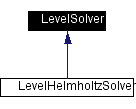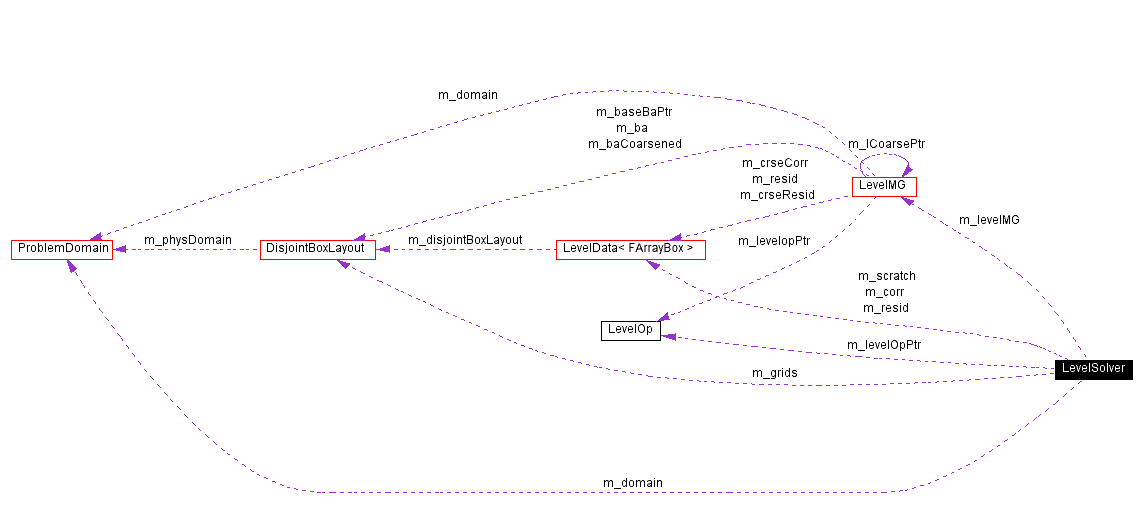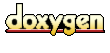Main Page   Namespace List   Class Hierarchy   Alphabetical List   Compound List   File List   Compound Members   File Members

# LevelSolver Class Reference

Solves elliptic equations on a level. More...

#include <LevelSolver.H>

Inheritance diagram for LevelSolver:[legend]
Collaboration diagram for LevelSolver:[legend]
List of all members.

## Public Methods

LevelSolver ()
virtual ~LevelSolver ()
LevelSolver (const DisjointBoxLayout &a_grids, const DisjointBoxLayout *a_baseGrids, const Box &a_domain, Real a_dxLevel, int a_nRefCrse, const LevelOp *const a_opin, int a_ncomp=1)
LevelSolver (const DisjointBoxLayout &a_grids, const DisjointBoxLayout *a_baseGrids, const ProblemDomain &a_domain, Real a_dxLevel, int a_nRefCrse, const LevelOp *const a_opin, int a_ncomp)
virtual void define (const DisjointBoxLayout &a_grids, const DisjointBoxLayout *a_baseGrids, const Box &a_domain, Real a_dxLevel, int a_nRefCrse, const LevelOp *const a_opin, int a_ncomp=1)
Full define function.

virtual void define (const DisjointBoxLayout &a_grids, const DisjointBoxLayout *a_baseGrids, const ProblemDomain &a_domain, Real a_dxLevel, int a_nRefCrse, const LevelOp *const a_opin, int a_ncomp=1)
Full define function.

bool isDefined () const
void clearMemory ()
void setDefaultValues ()
void levelSolveH (LevelData< FArrayBox > &phi, const LevelData< FArrayBox > &rhs, bool initializePhiToZero=true)
void levelSolve (LevelData< FArrayBox > &phi, const LevelData< FArrayBox > *phic, const LevelData< FArrayBox > &rhs, bool initializePhiToZero=true)
void setTolerance (Real a_tolerance)
set solution tolerance

void setVerbose (bool a_verbose)
void setMaxIter (int a_maxIter)
set max number multigrid vcycles to use in solution

## Protected Attributes

LevelMG m_levelMG
LevelOpm_levelOpPtr
bool m_isDefined
DisjointBoxLayout m_grids
ProblemDomain m_domain
LevelData< FArrayBoxm_resid
LevelData< FArrayBoxm_scratch
LevelData< FArrayBoxm_corr
int m_nRefCrse
Real m_dxLevel
int m_maxIter
Real m_tolerance
bool m_verbose

## Detailed Description

Solves elliptic equations on a level.

LevelSolver Solves elliptic equations on a level using multigrid.

## Constructor & Destructor Documentation

 LevelSolver::LevelSolver ( )
 Default constructor leaves LevelSolver undefined.

 virtual LevelSolver::~LevelSolver ( )  [virtual]

 LevelSolver::LevelSolver ( const DisjointBoxLayout & a_grids, const DisjointBoxLayout * a_baseGrids, const Box & a_domain, Real a_dxLevel, int a_nRefCrse, const LevelOp *const a_opin, int a_ncomp = 1 )
 Full constructor. Calls full define function.\ {\bf Inputs:} \ a_grids: Grid on which solution will be computed. \ a_baseGrids: pointer to next coarser grids. Should be == NULL if there is no coarser level. \ a_domain: domain of solution level \ a_dxLevel: grid spacing at solution level \ a_nRefCrse: refinement ratio between two levels. Not used if there is no finer level \ a_opin: operator L used in solution of Lphi=rho

 LevelSolver::LevelSolver ( const DisjointBoxLayout & a_grids, const DisjointBoxLayout * a_baseGrids, const ProblemDomain & a_domain, Real a_dxLevel, int a_nRefCrse, const LevelOp *const a_opin, int a_ncomp )
 Full constructor. Calls full define function.\ {\bf Inputs:} \ a_grids: Grid on which solution will be computed. \ a_baseGrids: pointer to next coarser grids. Should be == NULL if there is no coarser level. \ a_domain: domain of solution level \ a_dxLevel: grid spacing at solution level \ a_nRefCrse: refinement ratio between two levels. Not used if there is no finer level \ a_opin: operator L used in solution of Lphi=rho

## Member Function Documentation

 void LevelSolver::clearMemory ( )

 virtual void LevelSolver::define ( const DisjointBoxLayout & a_grids, const DisjointBoxLayout * a_baseGrids, const ProblemDomain & a_domain, Real a_dxLevel, int a_nRefCrse, const LevelOp *const a_opin, int a_ncomp = 1 )  [virtual]
 Full define function. Full define function.\ {\bf Inputs:} \ a_grids: Grid on which solution will be computed. \ a_baseGrids: pointer to next coarser grids. Should be == NULL if there is no coarser level. \ a_domain: domain of solution level \ a_dxLevel: grid spacing at solution level \ a_nRefCrse: refinement ratio between two levels. Not used if there is no finer level \ a_opin: operator L used in solution of Lphi=rho

 virtual void LevelSolver::define ( const DisjointBoxLayout & a_grids, const DisjointBoxLayout * a_baseGrids, const Box & a_domain, Real a_dxLevel, int a_nRefCrse, const LevelOp *const a_opin, int a_ncomp = 1 )  [virtual]
 Full define function. Full define function.\ {\bf Inputs:} \ a_grids: Grid on which solution will be computed. \ a_baseGrids: pointer to next coarser grids. Should be == NULL if there is no coarser level. \ a_domain: domain of solution level \ a_dxLevel: grid spacing at solution level \ a_nRefCrse: refinement ratio between two levels. Not used if there is no finer level \ a_opin: operator L used in solution of Lphi=rho

 bool LevelSolver::isDefined ( ) const

 void LevelSolver::levelSolve ( LevelData< FArrayBox > & phi, const LevelData< FArrayBox > * phic, const LevelData< FArrayBox > & rhs, bool initializePhiToZero = true )
 do a level solve on this level using multigrid uses inhomogeneous bcs at interfaces\ {\bf Inputs:} \ phi: initial guess at solution. phic: solution at next coarser level. should be == NULL if there is no coarser level. rhs: right-hand side. \ {\bf Outputs:} \ phi: solution.

 void LevelSolver::levelSolveH ( LevelData< FArrayBox > & phi, const LevelData< FArrayBox > & rhs, bool initializePhiToZero = true )
 do a level solve on this level using multigrid uses homogeneous bcs at coarse/fine interfaces \ {\bf Inputs:} \ phi: initial guess at solution. rhs: right-hand side. \ {\bf Outputs:} \ phi: solution.

 void LevelSolver::setDefaultValues ( )

 void LevelSolver::setMaxIter ( int a_maxIter )
 set max number multigrid vcycles to use in solution

 void LevelSolver::setTolerance ( Real a_tolerance )
 set solution tolerance

 void LevelSolver::setVerbose ( bool a_verbose )

## Member Data Documentation

 LevelData LevelSolver::m_corr [protected]

 ProblemDomain LevelSolver::m_domain [protected]

 Real LevelSolver::m_dxLevel [protected]

 DisjointBoxLayout LevelSolver::m_grids [protected]

 bool LevelSolver::m_isDefined [protected]

 LevelMG LevelSolver::m_levelMG [protected]

 LevelOp* LevelSolver::m_levelOpPtr [protected]

 int LevelSolver::m_maxIter [protected]

 int LevelSolver::m_nRefCrse [protected]

 LevelData LevelSolver::m_resid [protected]

 LevelData LevelSolver::m_scratch [protected]

 Real LevelSolver::m_tolerance [protected]

 bool LevelSolver::m_verbose [protected]

The documentation for this class was generated from the following file:
Generated on Thu Aug 29 11:07:45 2002 for Chombo&INS by1.2.16